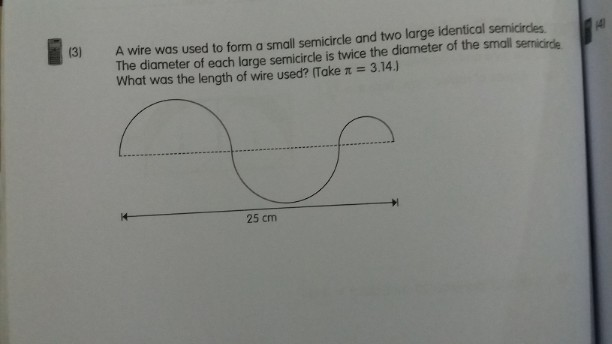# QuestionThe diameters of the all semi circles add up to 25 cm

Let the D of the small semi circle be D, and hence the bnigger semicircle will be 2D.

2D + 2D + D = 25
5D = 25
D = 5 , Smaller semicircle
2d = 10 , Bigger semicircle.

Length of wire will be the circumference of the 2 big semicricle plus the small semicircle.
= 2π X 5 + (2π X 2.5)/2
= 10π + 2.5π
= 12.5π = 39.25 cm ##

0 Replies 0 Likes